Next: Jutten-Hérault algorithm Up: Algorithms for ICA Previous: Introduction

## Preprocessing of the data

Some ICA algorithms require a preliminary sphering or whitening of the data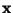, and even those algorithms that do not necessarily need sphering, often converge better with sphered data. (Recall that the data has also been assumed to be centered, i.e., made zero-mean.) Sphering means that the observed variableof Eq. (11) is linearly transformed to a variable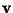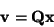(30)

such that the covariance matrix ofequals unity: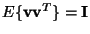. This transformation is always possible. Indeed, it can be accomplished by classical PCA [36,112,110,114]. In addition to sphering, PCA may allow us to determine the number of independent components (if m>n): if noise level is low, the energy ofis essentially concentrated on the subspace spanned by the nfirst principal components, with n the number of independent components in the model (11). Several methods exist for estimating the number of signals (here, independent components), see [146,94,144,11]. Thus this reduction of dimension partially justifies the assumption m=n that was made in Section 3, and will be retained here. After sphering we have from (11) and (30):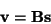(31)

where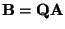is an orthogonal matrix, because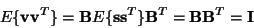Recall that we assumed that the independent components si have unit variance. We have thus reduced the problem of finding an arbitrary matrix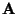in model (11) to the simpler problem of finding an orthogonal matrix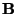. Onceis found, Eq. (31) is used to solve the independent components from the observedby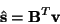(32)

It is also worthwhile to reflect why sphering alone does not solve the separation problem. This is because sphering is only defined up to an additional rotation: if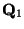is a sphering matrix, then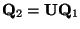is also a sphering matrix if and only if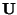is an orthogonal matrix. Therefore, we have to find the correct sphering matrix that equally separates the independent components. This is done by first finding any sphering matrix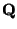, and later determining the appropriate orthogonal transformation from a suitable non-quadratic criterion.

In the following, we shall thus assume in certain sections that the data is sphered. For simplicity, the sphered data will be denoted by, and the transformed mixing matrix by, as in the definitions of Section 3. If an algorithm needs preliminary sphering, this is mentioned in the corresponding section. If no mention of sphering is made, none is needed.Next: Jutten-Hérault algorithm Up: Algorithms for ICA Previous: Introduction
Aapo Hyvarinen
1999-04-23